# Reversing.kr - Easy Keygen

## 1. Context

This is the next post of my reversing series following the previous post about Reversing.kr here.

This time, I’ll be working on the next challenge, Easy Keygen. Like the name implies, this type of challenge is for us to be able to reverse a key generation for a specific value to find the correct key!

## 2. Easy Keygen

First, like in Easy Crack, we should run the executable to see what to expect about its functionality.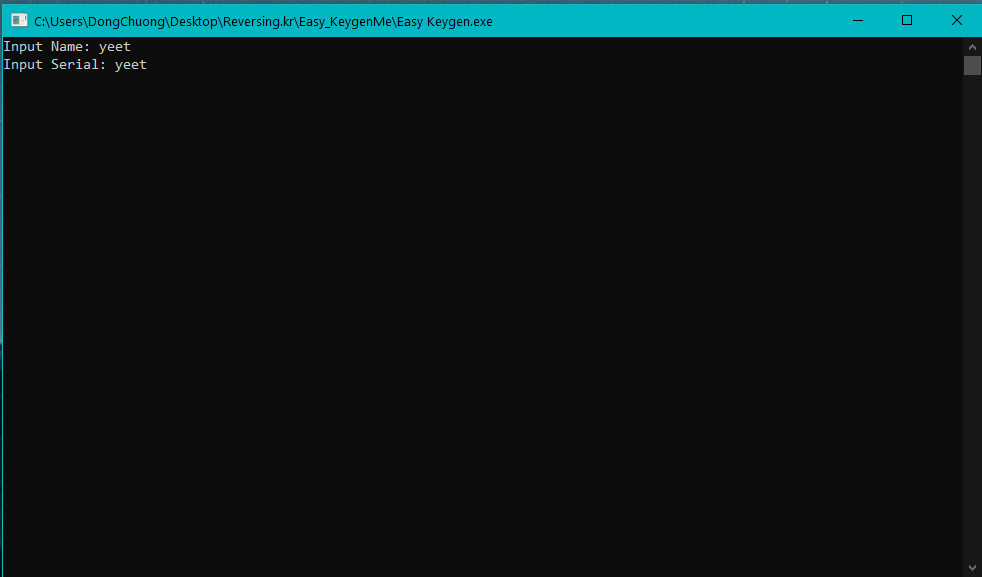Seems like the executable is just calling scanf to prompt us for an Input Name and an Input Serial. From viewing the note included in the challenge, we know that the Serial is 5B134977135E7D13.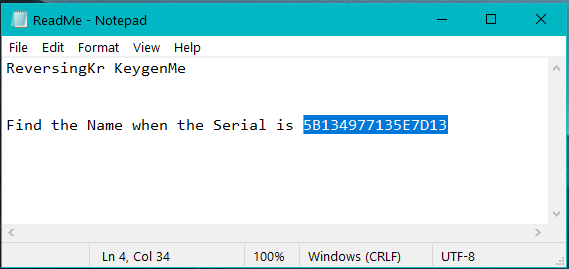For this keygen challenge, we must find the name corresponding to this serial in order to succeed! Let’s throw it into IDA and see what we get.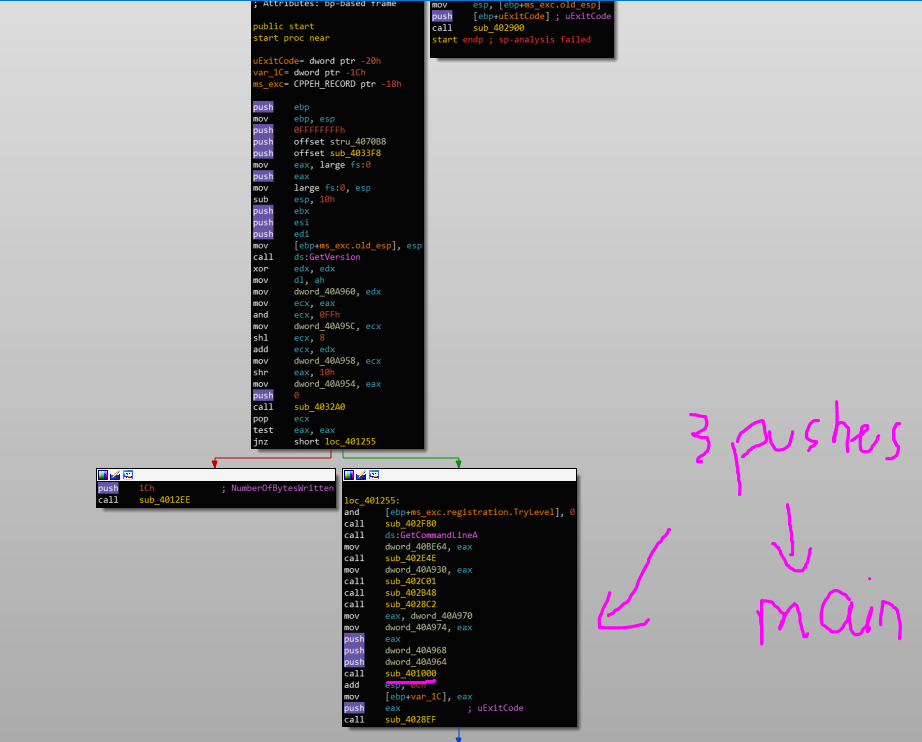When we first open IDA, it brings us right to the start address of the executable, which is the OEP (Original Entry Point). This is not the main method, most of the time, because the executable usually does some initial setup before making a call to main.

Usually, a trick that I use to find the main function is finding the one with 3 parameters being pushed onto the stack before the call. Here, we can see that it is sub_401000!

Let’s rename it to main and move on by clicking into this function.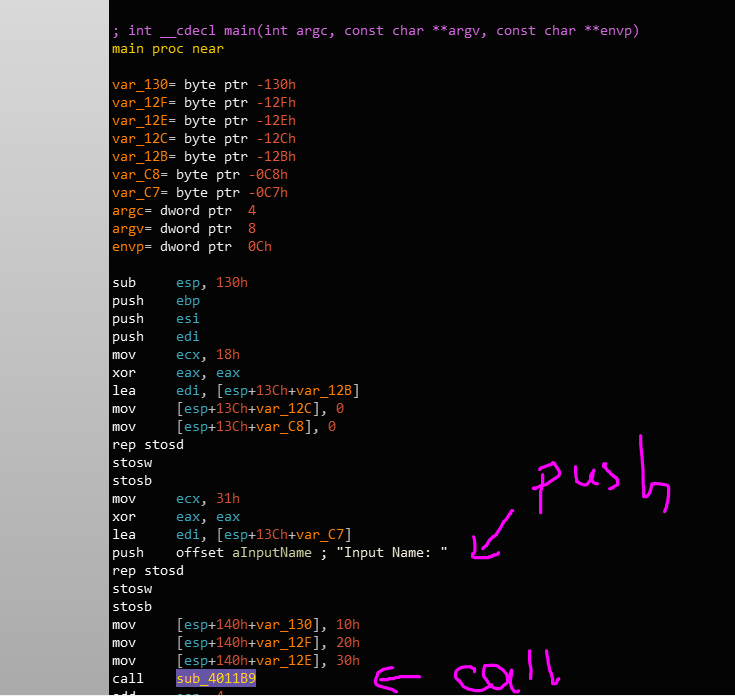The very first thing in main that we see is a call to sub_4011B9 with the string “Input Name: “ being pushed on the stack as its parameter.

Basically, the call looks like this in C++.

``````sub_4011B9("Input Name: ");
``````

From what we have seen from the original run, this function is most likely printf. However, if we want to make sure, we can use a debugger to check this. I’m using x32dbg for this, but any Windows debugger should work.

First, let’s put a breakpoint on the instruction at address 0x00401047 and run to it.

``````call sub_4011B9
``````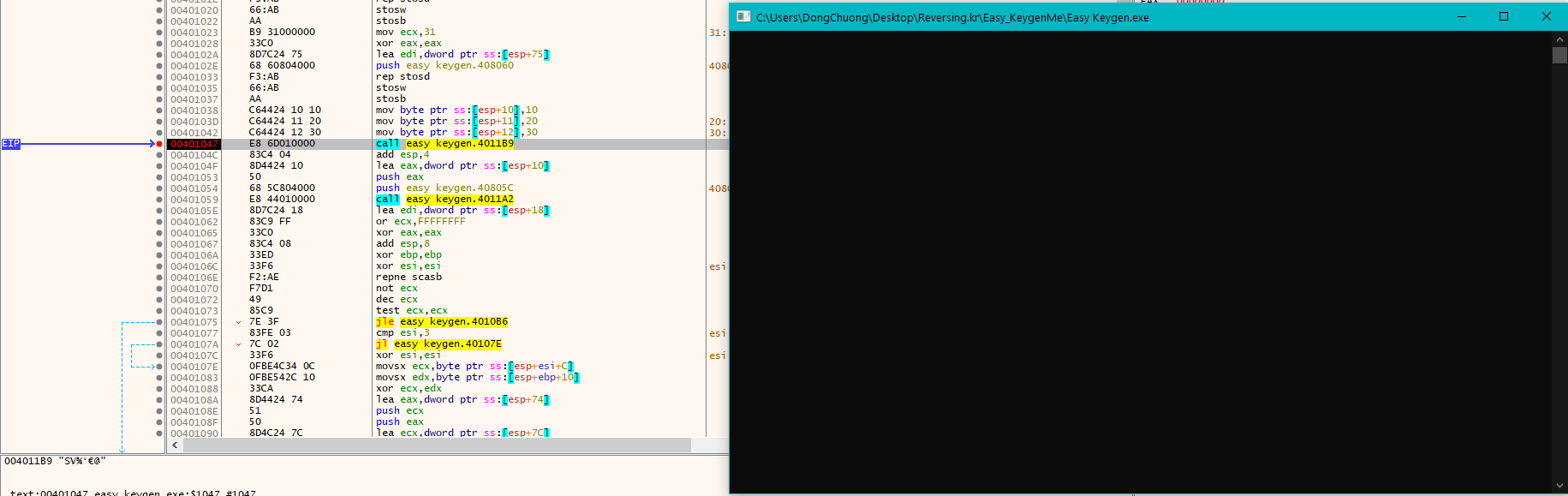Up until this call, nothing is being shown on the executable’s screen. If we hit F8 to step over this function call, we will see this.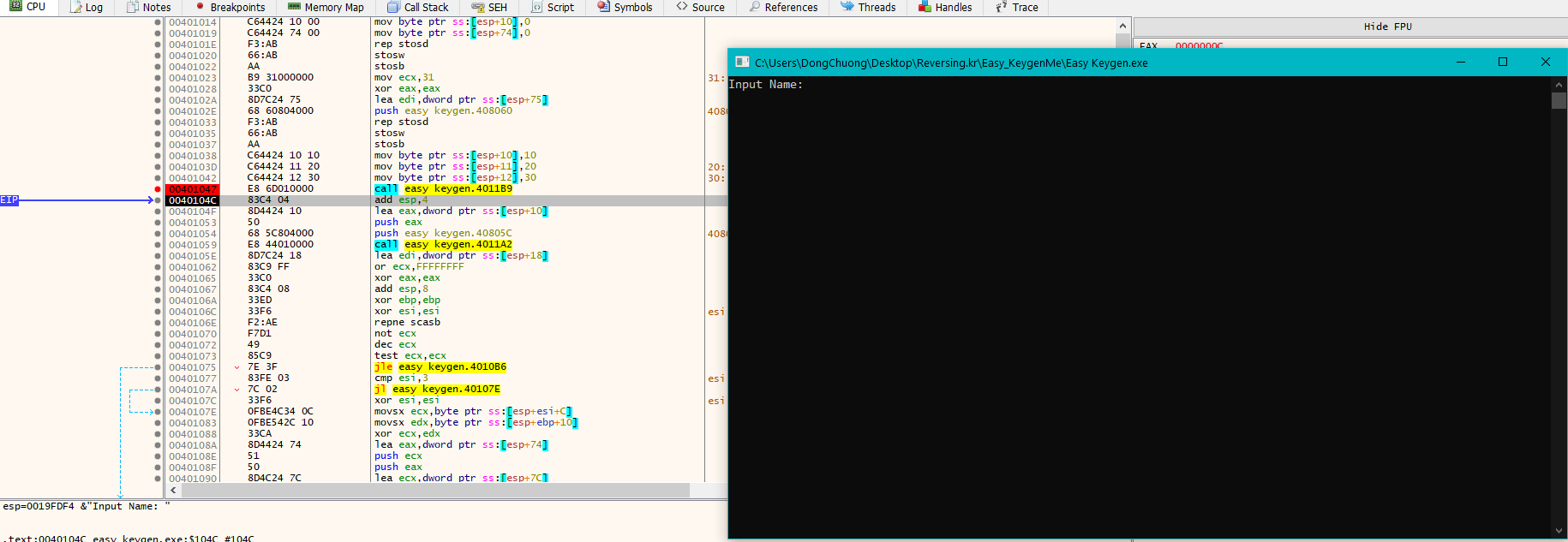This further confirms that sub_4011B9 is a printf function, so we can just rename it and move on!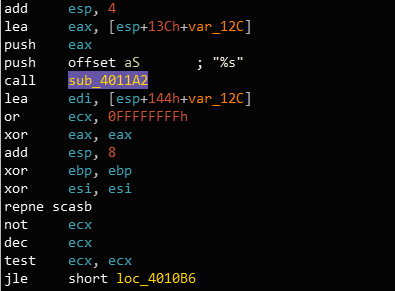Here, we see a call to sub_4011A2 with the string “%s” and the buffer var_12C as parameters. The call looks like this.

``````sub_4011A2("%s", var_12C);
``````

This is most likely to be a scanf call like we suspected, with the format string as the first parameter and the string buffer to write the string into as the second one.

We can again test this by executing this call using x32dbg and see what is in var_12C after the call.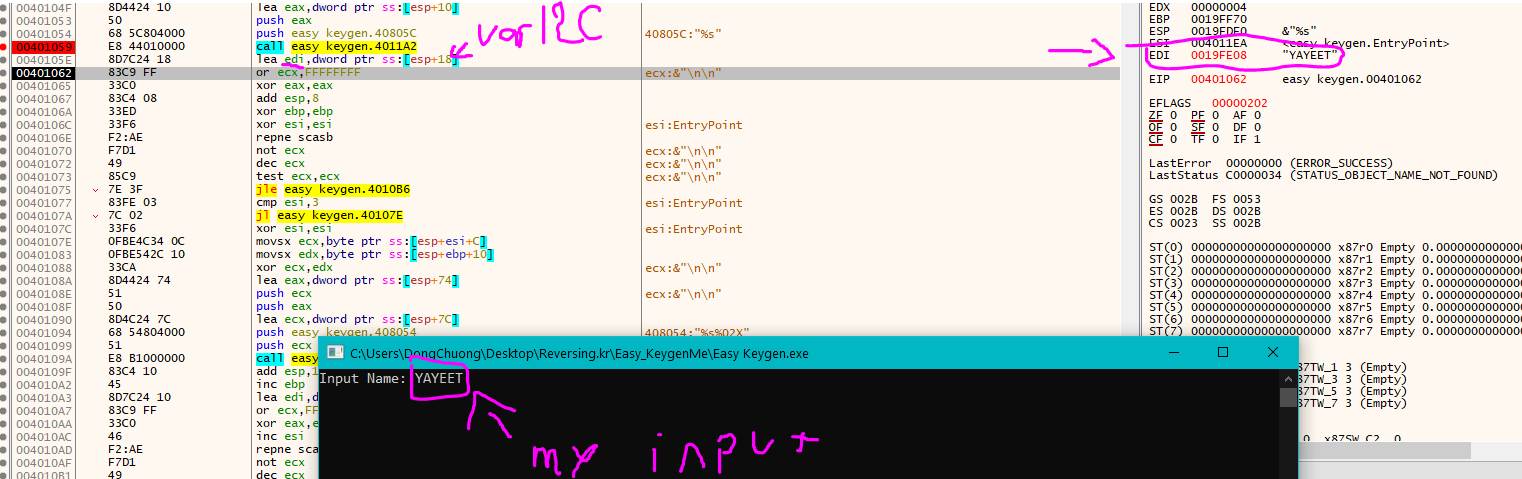When I give the string “YAYEET” as an input, the var_12C buffer is populated with that string as expected. We can see that the pointer to this string is being loaded into edi, so the string pointed to by edi is indeed our input string!

So now, we can be sure that sub_4011A2 is a scanf call. Let’s rename the function to scanf and var_12C to name_buffer and move on!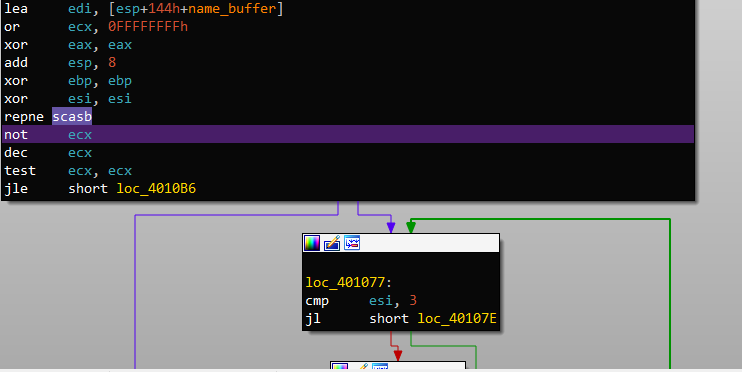Honestly, usually I tend to ignore these kind of assembly block of codes because I’m lazy, but since I’m writing a blog post about reversing, I guess I have to go deeper into it and fully explain what it means.Let’s break it down

``````lea     edi, [esp+144h+name_buffer]
or      ecx, 0FFFFFFFFh
xor     eax, eax
xor     ebp, ebp
xor     esi, esi
repne scasb
not     ecx
dec     ecx
test    ecx, ecx
``````

First, the pointer of name_buffer is loaded into edi. Next, ecx becomes 0xFFFFFFFF, and eax becomes 0. Finally, a call to repne scasb occurs.

In assembly, repne means repeat until not equals. scasb basically searches for the byte in eax(which is 0) in the memory that is starting in edi(which is name_buffer).

In this context, the call is repeated until we reach the end of the name_buffer string (because strings in C ends with a null-terminator). During this call, ecx is decremented for every byte comparison.

After the calls, ecx will be equal to `0xFFFFFFFF - len(name_buffer)`. By flipping all the bytes with a not call and decrement ecx, the finally value in ecx will be the length of name_buffer!! Here, this value is tested to see if it is 0 or not. You can read about the full repne scasb call here.

Let’s move on and see what happens when the length is not 0.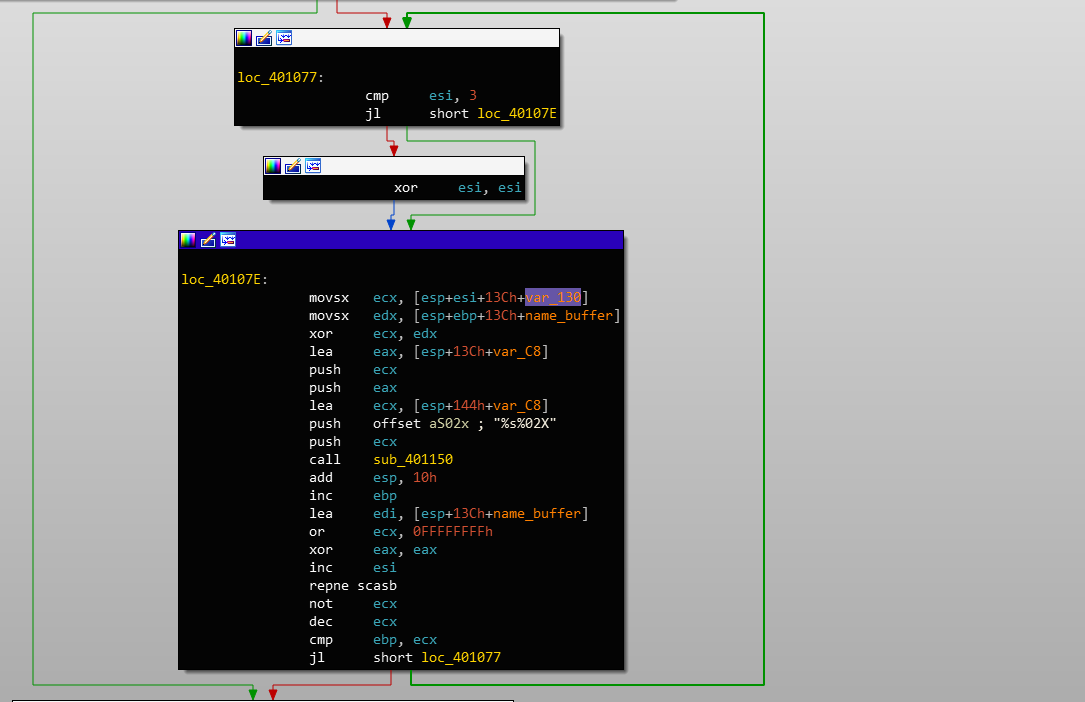First, we see that esi is being compared to 3, and if it is 3, esi is reset to 0! Therefore, during this loop, esi is updated in the range from 0 to 2 through every loop!

Next, we see the value of var_130 at index esi is loaded into ecx. But what is inside var_130?? If we look up a bit, we will see that it is just an array of byte `[0x10, 0x20, 0x30]`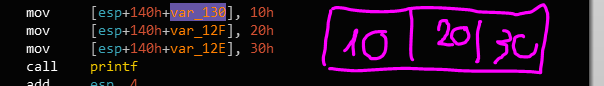Then, the value of name_buffer at index esi is loaded into edx and xor-ed with ecx. This value is then pushed onto the stack as the parameter for sub_401150. Let’s just run it dynamically and see if we can guess its functionality. I can dive into this rabbit hole and try to reverse this entire function, but it seems to be really long.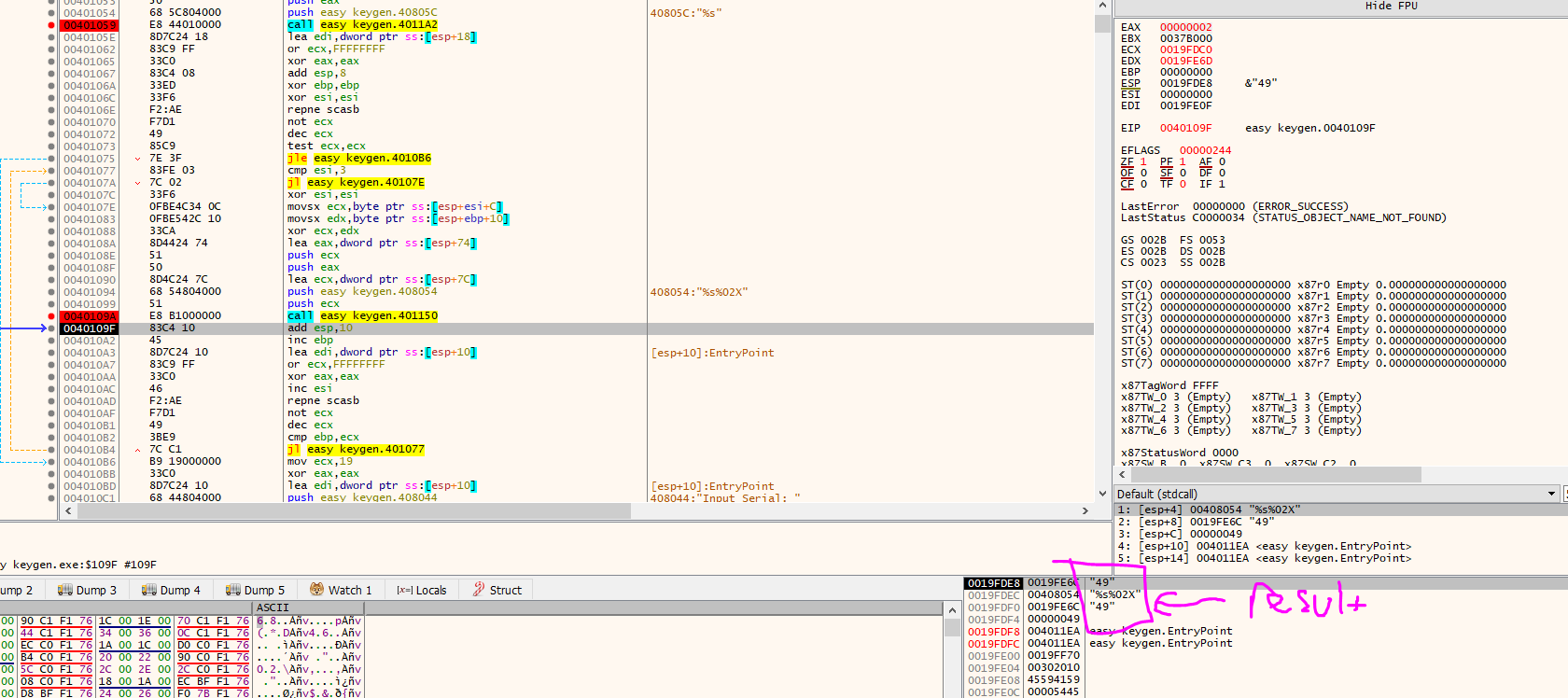When the input name_buffer is YAYEET, the first character is ‘Y’. The first value in var_130 is 0x10, and their xor result is 0x49. As we can see, this function converts the hex value 0x49 into the string “49” in ASCII and store it back into var_C8. Let’s rename the function to be convert_hex_to_string and var_C8 into hex_name_buffer.

This loop will go until we reach the end of name_buffer, and the XOR-encrypted name will be stored inside hex_name_buffer.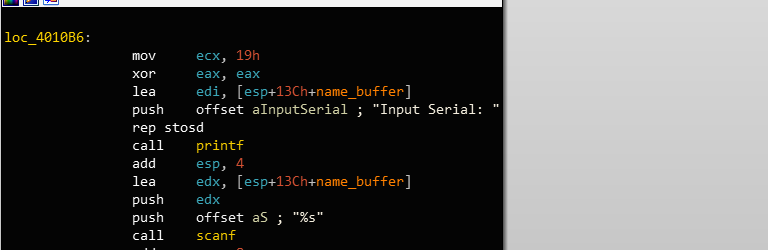Next, we see a similar piece of code with printf and scanf. This time, the Serial is being input into name_buffer.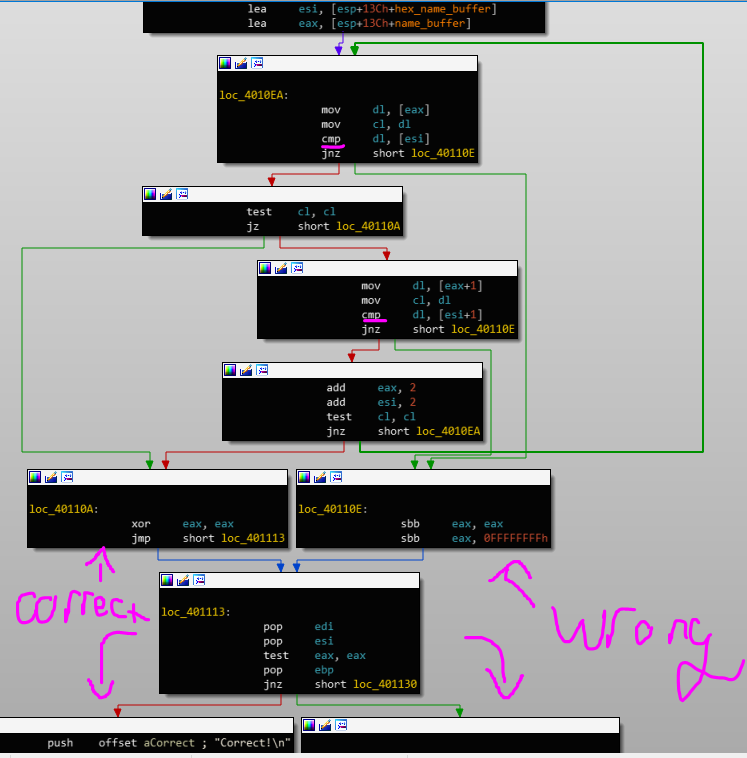Here, we see a familiar loop to compare strings like in my previous post! Therefore, hex_name_buffer is being compared with the Serial number, and if they match, we get a correct message!!

So now, we know that in order to get the correct name for the serial number, we have to reverse the XOR algorithm earlier.

Here is a quick Python script that I wrote to extract the correct name!

``````serial = "5B134977135E7D13"
key = [0x10, 0x20, 0x30]

real_serial = []
i = 0
while i < len(serial) - 1:
real_serial.append(int('0x' + serial[i:i + 2], 16))
i += 2

name = ''
i = 0
for each in real_serial:
name += chr(each ^ key[i])
i = (i + 1) % 3
print(name)
``````

Running this script, we will get K3yg3nm3 as the correct name for this Serial number. If we type it in, we will see this in the executable.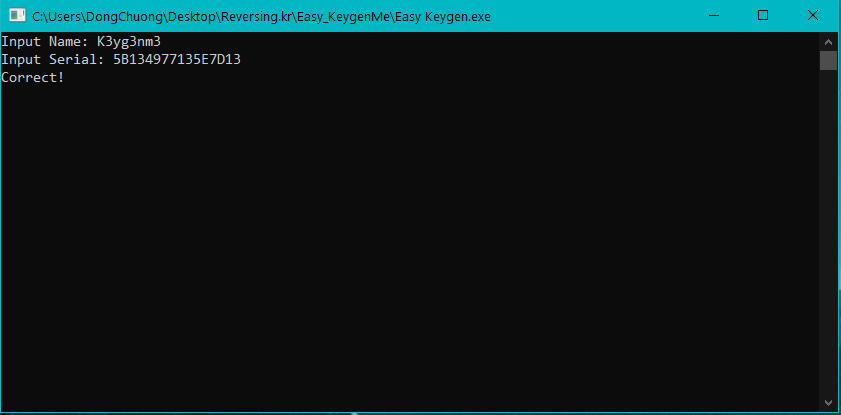## 3. Recap

This challenge is pretty straightforward. It basically boils down to a XOR-encryption and the conversion between a hex value into its string form!